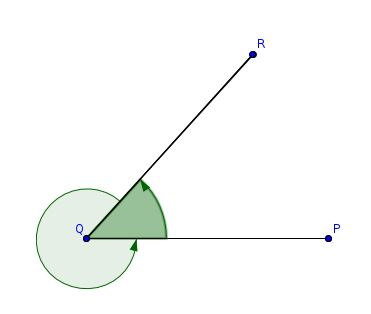Welcome guest
You're not logged in.
276 users online, thereof 0 logged in

## Definition: 1.09: Angle, Rectilinear, Vertex, Legs

### (Definition 9 from Book 1 of Euclid’s “Elements”)

And when the lines containing the plane angle are straight then the angle is called rectilinear.

### Modern Definition

Let $$\overline{P Q}$$ and $$\overline{Q R}$$ be two given segments  1, which meet in the point $$Q$$. The inclination of $$\overline{PQ}$$ and $$\overline{QR}$$, is called a rectilinear angle, or simply an angle. The point $$Q$$ is called the vertex of the angle and the segments are called its legs.

1 This definition holds when we replace the term “segments” by “straight lines” or “rays”.

### Please note the following important notation convention used in this adaptation of Euclid’s “Elements”:

In order to avoid ambiguities in notation, given a drawing of an angle, we think of an angle as an oriented rotation of its legs around its vertex, and always assume that this rotation is counter clockwise oriented. With this in our mind, in this adaptation of Euclid’s “Elements”, we always denote all angles beginning with the leg, where the thought rotation starts, and ending with the leg, where the thought rotation stops! In order to demonstrate this principle, consider the following figure:To denote the smaller angle, we write $$\angle{PQR}$$, because the leg $$\overline{PQ}$$ is being rotated around the vertex $$Q$$ to the leg $$\overline{QR}$$. To denote the bigger angle, we write $$\angle{RQP}$$, because the leg $$\overline{RQ}$$ is being rotated around the vertex $$Q$$ to the leg $$\overline{QP}$$.

## 3.Definition: Supplemental Angles

### CC BY-SA 4.0

 Callahan, Daniel: “Euclid’s ‘Elements’ Redux”, http://starrhorse.com/euclid/, 2014